### Home > MC2 > Chapter 5 > Lesson 5.1.3 > Problem5-34

5-34.
1. Sketch each collection of tiles below. Name the collection using a simpler algebraic expression, if possible. If it is not possible to simplify the expression, explain why not. 5-34 b&c HW eTool (CPM). Homework Help ✎

1. (–x) + 7 + 4x + x + (–3) + (–3x)

2. Seven plus three times a number, plus three minus three times the number.

3. Two groups of a number plus three.

4. 7 + 4x2 + 5x

5. x2 + x2 + (–5) + 2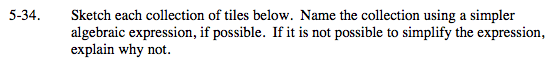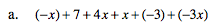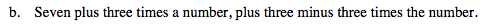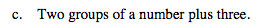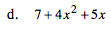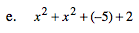Explore the problem with the eTool below.
Click the link at right for the full version of the eTool: MC2 5-34 HW eTool